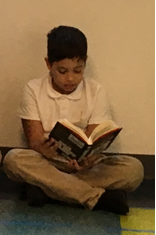# Multi-Digit Division

## Objective

Solve two-step word problems, including those involving interpreting the remainder, and assess the reasonableness of answers.

## Common Core Standards

### Core Standards

?

• 4.NBT.B.6 — Find whole-number quotients and remainders with up to four-digit dividends and one-digit divisors, using strategies based on place value, the properties of operations, and/or the relationship between multiplication and division. Illustrate and explain the calculation by using equations, rectangular arrays, and/or area models.

• 4.OA.A.3 — Solve multistep word problems posed with whole numbers and having whole-number answers using the four operations, including problems in which remainders must be interpreted. Represent these problems using equations with a letter standing for the unknown quantity. Assess the reasonableness of answers using mental computation and estimation strategies including rounding.

?

• 4.NBT.B.4

• 4.NBT.B.5

• 3.OA.D.8

## Criteria for Success

?

1. Make sense of a three-act task and persevere in solving it (MP.1).
2. Solve two-step word problems involving all four operations (MP.4).
3. Interpret the remainder in the context of a problem (MP.1).
4. Assess the reasonableness of an answer (MP.1).

## Tips for Teachers

?

• As noted in Units 1 and 2, it isn’t especially clear whether students are expected to interpret, understand, and/or write one equation that represents a two- or multi-step word problem. As the Progressions state, “some problems might easily be represented with a single equation, and others will be more sensibly represented by more than one equation or a diagram and one or more equations” (OA Progression, p. 29). Thus, it is left to the teacher to decide what “easily” and “sensibly” means for your students, and when to make the transition to representing some two- and multi-step word problems with one step of representation/one equation, which you could do here or wait for subsequent lessons and units to do so. As some general guidance, it seems reasonable to expect every student to be able to write an equation for all one-step problems, two-step problems where the unknown is isolated on one side of the equal sign in the equation without needing to manipulate it, and some multi-step problems with the same parameters by the end of the year. For example, see Question #2 in New York State Testing Program Mathematics Common Core Sample Questions Grade 4, Question #5c in Spring 2016 Grade 4 Mathematics Test by MCAS, and Question #11 in Math Spring 2017 Grade 4 Released Items by PARCC.
• “To compute and interpret remainders in word problems (4.OA.A.3), students must reason abstractly and quantitatively (MP.2), make sense of problems (MP.1), and… search for the structure (MP.7) in problems with similar interpretations of remainders” (PARCC Model Content Frameworks, Mathematics, Grade 3—11).
• This lesson provides an opportunity to connect two domains in the grade, 4.OA and 4.NBT, since students will be solving two-step word problems involving addition, subtraction, multiplication, and division and assessing the reasonableness of their answers using rounding, thus connecting standards 4.OA.3, 4.NBT.3, 4.NBT.4, 4.NBT.5, and 4.NBT.6.
• Let students work on each problem in the Problem Set independently and circulate to see whether students are solving correctly. If not, come back together to discuss how/what to draw on a tape diagram, then allow them to try again on their own. Encourage students to use a strategy of their choice to assess the reasonableness of their answer.

#### Remote Learning Guidance

This lesson does not have any identified priority Anchor Tasks, but students should complete the Problem Set independently rather than skipping the lesson entirely. Find more guidance on adapting our math curriculum for remote learning here.

#### Fishtank Plus

• Problem Set
• Student Handout Editor
• Vocabulary Package

?

### Problem 1

Act 1: Look at the following image -How long will it take him to finish the book?

### Problem 2

Act 2: Use the following information to solve -

• Diary of a Wimpy Kid is 221 pages long.

### Problem 3

Act 3: Reveal the answer -

It will take Joao 23 blocks of independent reading to finish the book.

### Problem 4

Act 4 (the sequel) -

Joao loves The Diary of a Wimpy Kid and convinces Ms. Glynn to buy as many as she can for his classmates to read. Ms. Glynn has $150 left in her classroom budget to buy them. Each book costs$8. How many copies can she get?

## Problem Set & Homework

#### Discussion of Problem Set

• How did you interpret the remainder in #2, 3, 5, 6, 7, and 8?
• What equation did you write in #4, Part A? How did you use your equation to help you with Part B?
• What equation did you write in #7? How did you use your equation to help you find the number of tables needed for all of the students? Is the solution to that equation the answer, or does it still require some interpretation?
• How many tickets could Jalen have sold? Why is there more than one correct answer?
• About how many empty parking spaces will the garage have in #9, Part C? Can you use that rounded answer to assess the reasonableness of your answer? Why or why not?

?

Solve. Show or explain your work.

A photographer has 591 photos of animals and 234 photos of plants. He wants to put all of the photos into photo books. Each page of the photo book holds 8 photos. What is the fewest number of pages he could use in the photo books?

#### References

PARCC Released Items Spring Operational 2015 Grade 4 End of Year Released ItemsQuestion Stem #21

### Mastery Response

?